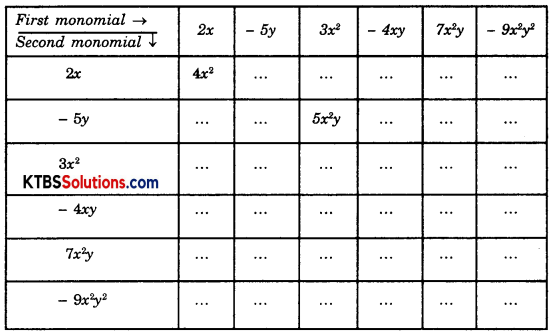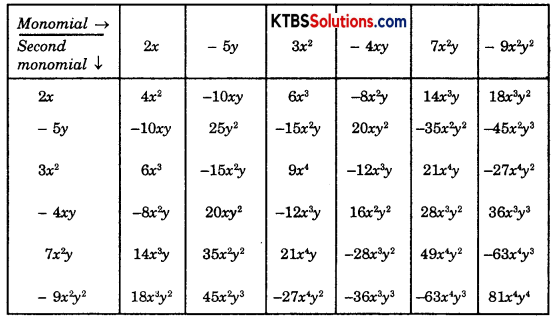# KSEEB Solutions for Class 8 Maths Chapter 9 Algebraic Expressions and Identities Ex 9.2

You can Download KSEEB Solutions for Class 8 Maths Chapter 9 Algebraic Expressions and Identities Ex 9.2 Questions and Answers to help you to revise the complete syllabus.

## KSEEB Solutions for Class 8 Maths Chapter 9 Algebraic Expressions and Identities Ex 9.2

Question 1.
Find the product of the following pairs of monomials.
(i) 4, 7p
(ii) -4, 7p
(iii) -4p, 7pq
(iv) 4p3, -3p
(v) 4p, 0
Solution:
(i) 4, 7p = 28p
(ii) -4, 7p = -28p2
(iii) -4p, 7pq = -28p2q
(iv) 4p3, -3p = -12p2
(v) 4p, 0 = 0Question 2.
Find the areas of rectangles with the following pairs of monomials as their lengths and breadths respectively.
(p, q); (10m, 5n); (20x2, 5y2); (4x, 3x2); (3mn, 4np)
Solution:
Since the area of rectangle is the product of length × Breadth
(i) p × q = pq
(ii) 10m × 5n = 50mn
(iii) 20x2 × 5y2 = 100x2y2
(iv) 4x × 3x2 = 12x3
(v) 3mn × 4np = 12mn2p

Question 3.
Complete the table of products.Solution:Question 4.
Obtain the volume of rectangular boxes with the following length, breadth, and height respectively.
(i) 5a, 3a2, 7a4
(ii) 2p, 4q, 8r
(iii) xy, 2x2y, 2xy2
(iv) a, 2b, 3c
Solution:
Volume of rectangular boxes is length × breadth × height
(i) 5a, 3a2, 7a4
= 5 × 3 × 7 × a1+2+4
= 105a7

(ii) 2p, 4q, 8r
= 2p, 4q, 8r
= 2 × 4 × 8pqr
= 64pqr(iii) xy, 2x2y, 2xy2
= 1 × 2 × 2 × xy × x2y × xy2
= 4x4y4

(iv) a × 2b × 3c
= 1 × 2 × 3 × abc
= 6abc

Question 5.
Obtain the product of
(i) xy, yz, zx
(ii) a, -a2, a3
(iii) 2, 4y, 8y2, 16y3
(iv) a, 2b, 3c, 6abc
(v) m, -mn, mnp
Solution:
(i) xy × yz × zx
= x2y2z2

(ii) ax – a2 × a3
= 1 × 1 × 1 × a × a2 × a3
= 1 × a6
= -a6

(iii) 2 × 4y × 8y2 × 16y3
= 2 × 4 × 8 × 16 × y × y2 × y3
= 102y6(iv) a × 2b × 3c × 6abc
= 1 × 2 × 3 × 6 × a × b × c × abc
= 36a2b2c2

(v) m × -mn × mnp
= 1 × -1 × 1 × m × -mn × mnp
= -1m2n2p